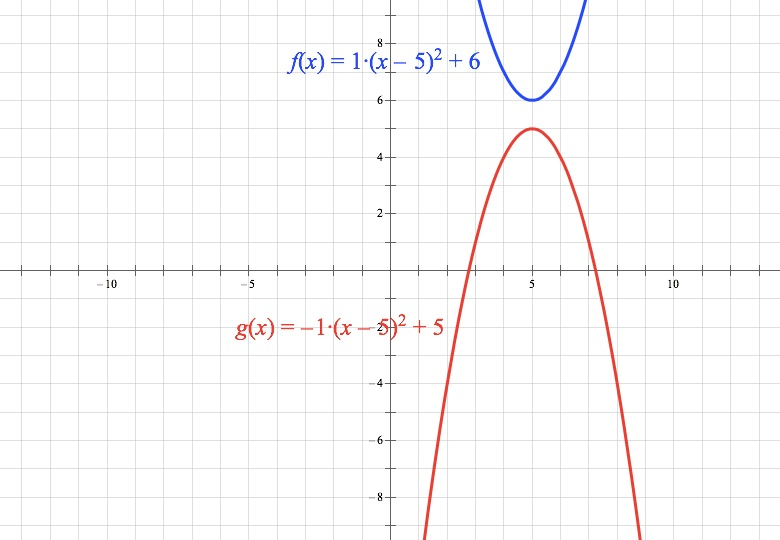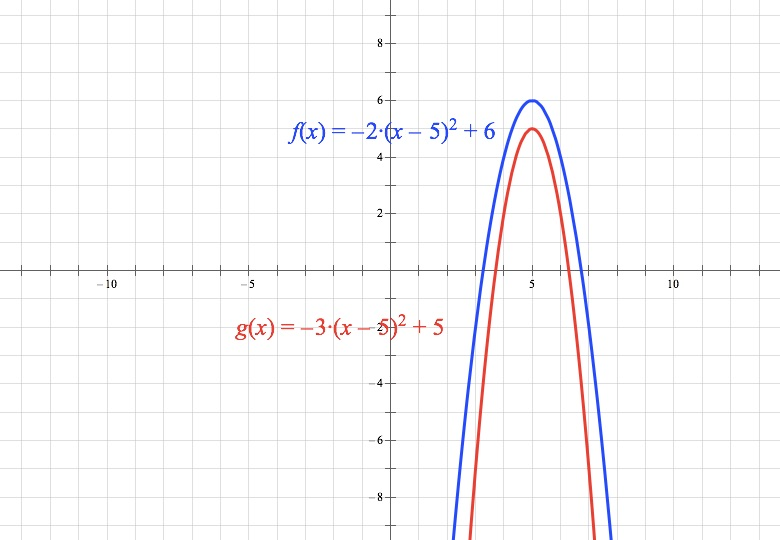In PWN p. 159 (p. 157 later printing) #8
In the xy-plane, where a and b are constants, the graphs …

The question does not specify that a and b are positive values. If one or both were negative, wouldn’t that change the answer?Good question! It turns out, even though my solution assumes a and b are positive, that they don’t need to be. You have two parabolas with vertices on the x = 5 line, one higher than the other. As long as the leading coefficient of the higher one is greater than or equal to the leading coefficient of the lower one, the parabolas will not intersect. C is the answer.

Let’s look at the cases you’re asking about, where one or both of the coefficients is negative. First, say a = 1 and b = –1. In that case, ab, and one variable is negative.We’re OK there—obviously those parabolas won’t ever intersect.

In the case where both are negative but ab, it turns out we’re STILL fine. Let’s say a = –2 and b = –3. It’s still true that ab, and both coefficients are negative.Those also won’t ever intersect.

What’s going on here is that as long as ab and both signs are the same, the absolute value of the leading coefficient of the inner parabola will be higher—the inner parabola will be skinnier than the outer parabola!

That’s a lot to think about on one question though—maybe in the next edition I should just make them positive constants!